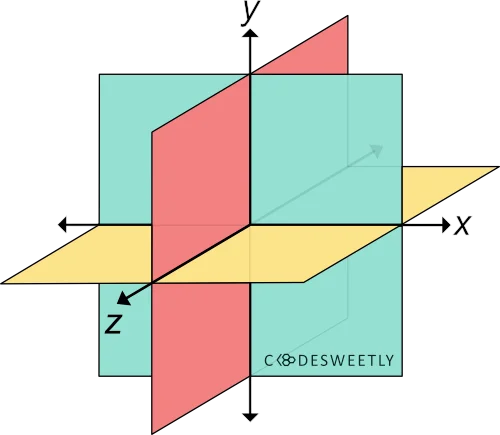# CSS rotate3d() Function – How to Rotate Elements in 3D

rotate3d() transforms an element by rotating it three-dimensionally around the x-, y-, and z-axis.A three-dimensional Cartesian coordinate system showing the X-, Y-, and Z-axis

## Syntax of the CSS `rotate3d()` Function​

`rotate3d()` accepts four arguments. Here is the syntax:

``element {  transform: rotate3d(x, y, z, angle);}``

Note the following:

• The `x`, `y`, and `z` arguments are numbers specifying the x-, y-, and z-coordinates.
• The coordinates are the axis around which the element will rotate.
• The `angle` argument specifies the element's angle of rotation.
• `angle` can be in degree, gradian, radian, or turn.
• An `angle` argument consists of a number followed by the unit you wish to use—for instance, `45deg`.

## Examples of the CSS `rotate3d()` Function​

Below are some examples of how the CSS `rotate3d()` function works.

### How to do a 70-degree rotation around the Z-axis​

``img {  transform: rotate3d(0, 0, 1, 70deg);  width: 80%;}``

Try it on StackBlitz

The snippet above used the `rotate3d()` function to specify a seventy-degree (70⁰) rotation for the image around the Z-axis.

### How to do a 70-degree rotation around the X-, Y-, and Z-axis​

``img {  transform: rotate3d(1, 1, 1, 70deg);  width: 80%;}``

Try it on StackBlitz

The snippet above used the `rotate3d()` function to specify a seventy-degree (70⁰) rotation for the image around the x-, y-, and z-axis.

## Overview​

This article discussed what a CSS `rotate3d()` function is. We also used examples to see how it works.